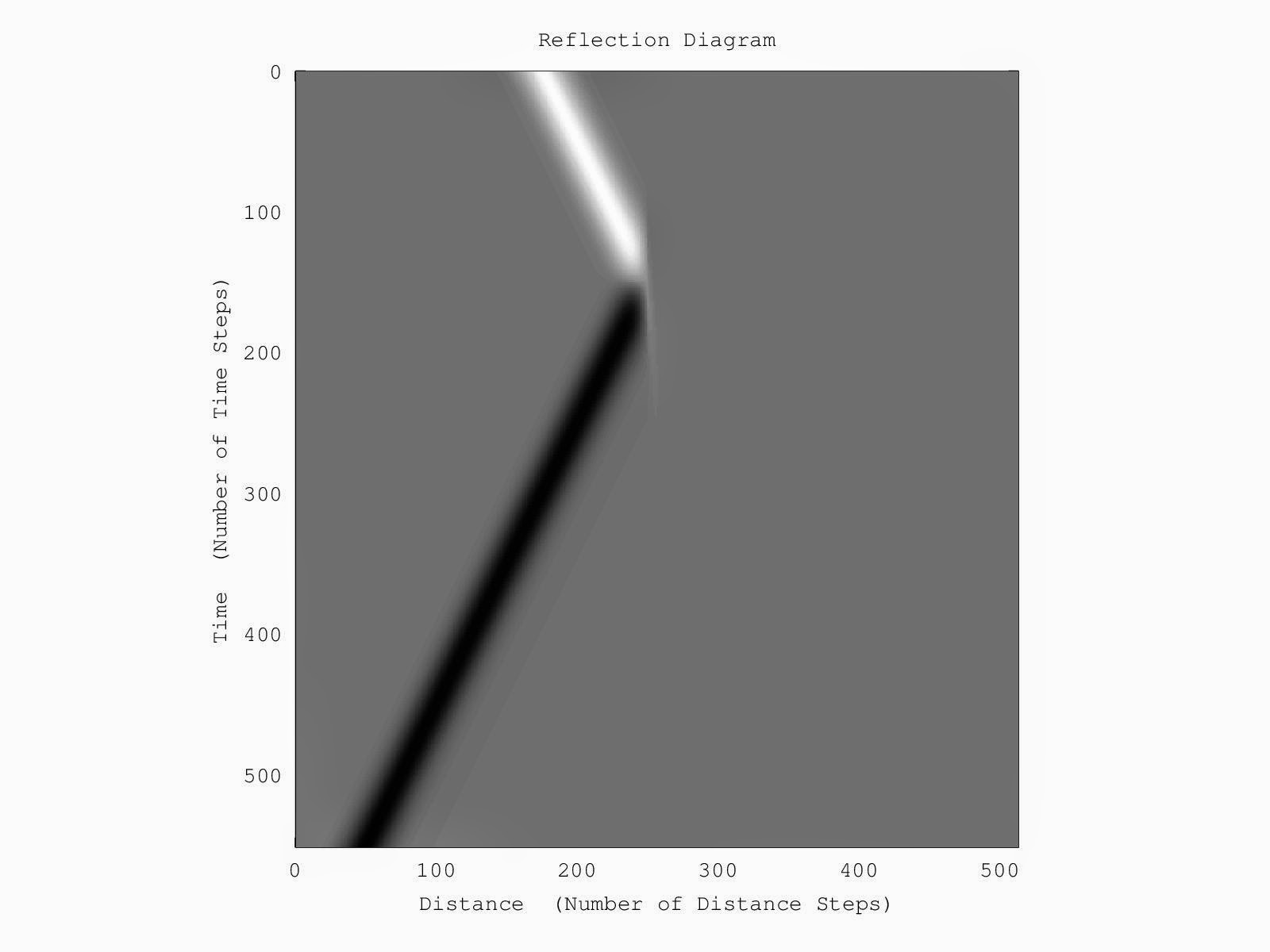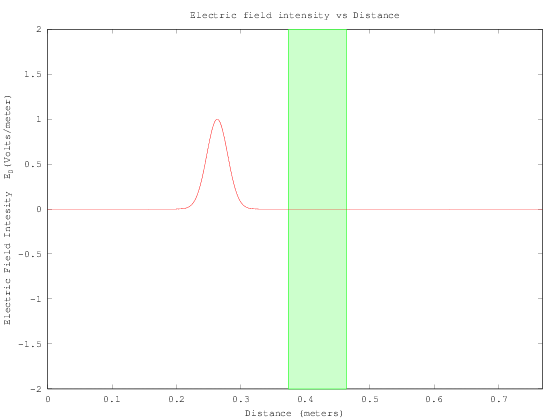## Saturday, July 5, 2014

### I hope you find this blog enjoyable and helpful. Support it with a small donation.

I've been toying around with some old code from uni that models a propagating electromagnetic field, and I thought I'd do some simulations to illustrate how fields propagate and interact with matter.  Usually I'd include the code I used to do the simulations, but as it was for an assignment I don't know if I should.  Do your own homework kids :-).  However if you need more info don't be afraid to ask.

Each simulation is of a gaussian pulse in free space propagating from the left impacting a lossy dielectric shown in green.  The simulations are in 1D making thing easy and are analogous to a signal propagating in a transmission line.

The relative permittivity of the material in all simulations is 7, so let's say it's rubber.  For the first simulation I've set the conductivity to 0 S/m, this means it's lossless.

The are several things to observe in the animation below.  The initial interaction with the material creates a transmitted and reflected wave.  The reflected portion is inverted, this happens when an electromagnetic wave crosses an interface from a low to high dielectric material.  It can also be seen that the speed of the wave decreases as it travels through the material.  Anyone familiar with transmission lines would be familiar with this and know it as velocity factor.   The velocity factor is equal to the inverse square root of the relative dielectric constant.  In this case that's equal to 37.8 percent.  The wave continues on and is transmitted and reflected again.  This time the reflection isn't inverted, this is because the wave is travelling from a high dielectric material to a low one. The transmitted portion of this wave increases at this point, which seems counter intuitive, but you have to remember we're looking at the electric field, not the power flow which is also related to the dielectric constant of the material.Electromagnetic wave impacting a lossless dielectric

The reflection diagram helps to visualise the propagation of the wave.  White indicates positive waves, while black indicates negative waves.  Time progresses as you go down the graph.  The change in velocity of the wave in the dielectric medium is evident here.Electromagnetic wave impacting a lossless dielectric

For this simulation I have set the conductivity to 10 S/m.  It's not a metal but it's conductive enough to demonstrate some things.  To give a point of comparison, sea water is 4.8 S/m.   Basically the wave is completely reflected.  A small portion of it is transmitted but it quickly dissipates.  It also gives me a chance to tell you how I remember how a wave is reflected from a conductor.  Electric field is analogous to voltage, and because a conductor effectively short circuits the wave, the electric field at the interface has to be zero.  If the material in the simulation was a perfect electric conductor this is the behaviour we would see.  For the electric field to be zero the reflected wave has to be the negative of the incident wave.  When these are added you end up with zero electric field at the boundary.  So the electric field of a wave reflected from a conductor is inverted.Electromagnetic wave impacting a high loss dielectricElectromagnetic wave impacting a high loss dielectric

For the final simulation I've set the conductivity to 0.15 S/m.  Lets say it's a conductive rubber of some sort.  The simulation allows us to see the how the wave is attenuated as it travels through the material.  Some does make it through, but it's reduced in magnitude.Electromagnetic wave impacting a lossy dielectricElectromagnetic wave impacting a lossy dielectric